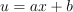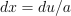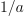/NumPy 1.17

# numpy.polynomial.legendre.legint

`numpy.polynomial.legendre.legint(c, m=1, k=[], lbnd=0, scl=1, axis=0)` [source]

Integrate a Legendre series.

Returns the Legendre series coefficients `c` integrated `m` times from `lbnd` along `axis`. At each iteration the resulting series is multiplied by `scl` and an integration constant, `k`, is added. The scaling factor is for use in a linear change of variable. (“Buyer beware”: note that, depending on what one is doing, one may want `scl` to be the reciprocal of what one might expect; for more information, see the Notes section below.) The argument `c` is an array of coefficients from low to high degree along each axis, e.g., [1,2,3] represents the series `L_0 + 2*L_1 + 3*L_2` while [[1,2],[1,2]] represents ```1*L_0(x)*L_0(y) + 1*L_1(x)*L_0(y) + 2*L_0(x)*L_1(y) + 2*L_1(x)*L_1(y)``` if axis=0 is `x` and axis=1 is `y`.

Parameters: `c : array_like` Array of Legendre series coefficients. If c is multidimensional the different axis correspond to different variables with the degree in each axis given by the corresponding index. `m : int, optional` Order of integration, must be positive. (Default: 1) `k : {[], list, scalar}, optional` Integration constant(s). The value of the first integral at `lbnd` is the first value in the list, the value of the second integral at `lbnd` is the second value, etc. If `k == []` (the default), all constants are set to zero. If `m == 1`, a single scalar can be given instead of a list. `lbnd : scalar, optional` The lower bound of the integral. (Default: 0) `scl : scalar, optional` Following each integration the result is multiplied by `scl` before the integration constant is added. (Default: 1) `axis : int, optional` Axis over which the integral is taken. (Default: 0). New in version 1.7.0. `S : ndarray` Legendre series coefficient array of the integral. ValueError If `m < 0`, `len(k) > m`, `np.ndim(lbnd) != 0`, or `np.ndim(scl) != 0`.

#### Notes

Note that the result of each integration is multiplied by `scl`. Why is this important to note? Say one is making a linear change of variablein an integral relative to `x`. Then, so one will need to set `scl` equal to- perhaps not what one would have first thought.

Also note that, in general, the result of integrating a C-series needs to be “reprojected” onto the C-series basis set. Thus, typically, the result of this function is “unintuitive,” albeit correct; see Examples section below.

#### Examples

```>>> from numpy.polynomial import legendre as L
>>> c = (1,2,3)
>>> L.legint(c)
array([ 0.33333333,  0.4       ,  0.66666667,  0.6       ]) # may vary
>>> L.legint(c, 3)
array([  1.66666667e-02,  -1.78571429e-02,   4.76190476e-02, # may vary
-1.73472348e-18,   1.90476190e-02,   9.52380952e-03])
>>> L.legint(c, k=3)
array([ 3.33333333,  0.4       ,  0.66666667,  0.6       ]) # may vary
>>> L.legint(c, lbnd=-2)
array([ 7.33333333,  0.4       ,  0.66666667,  0.6       ]) # may vary
>>> L.legint(c, scl=2)
array([ 0.66666667,  0.8       ,  1.33333333,  1.2       ]) # may vary
```

© 2005–2019 NumPy Developers
Licensed under the 3-clause BSD License.
https://docs.scipy.org/doc/numpy-1.17.0/reference/generated/numpy.polynomial.legendre.legint.html